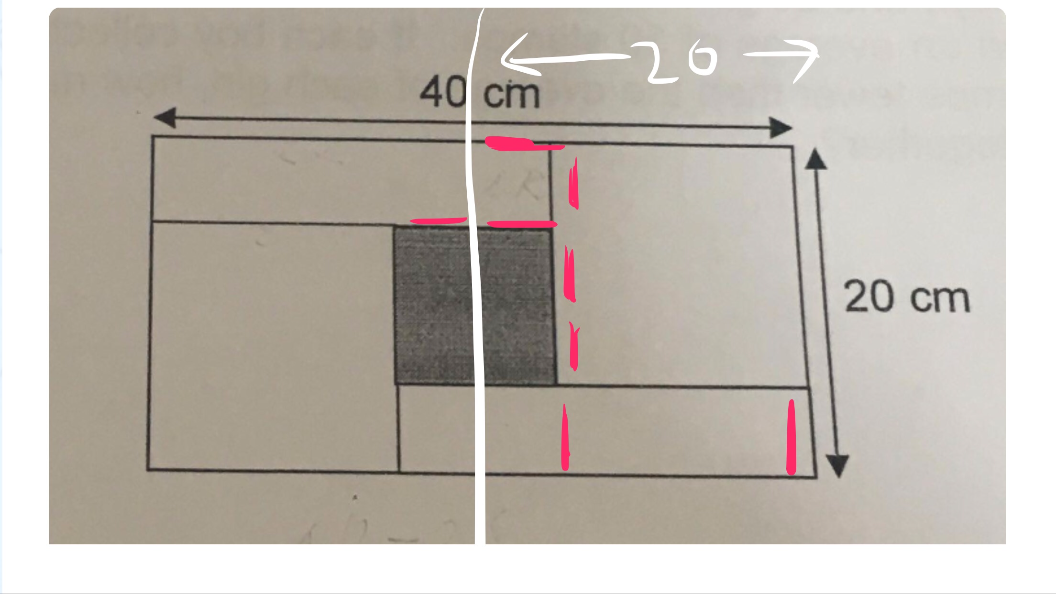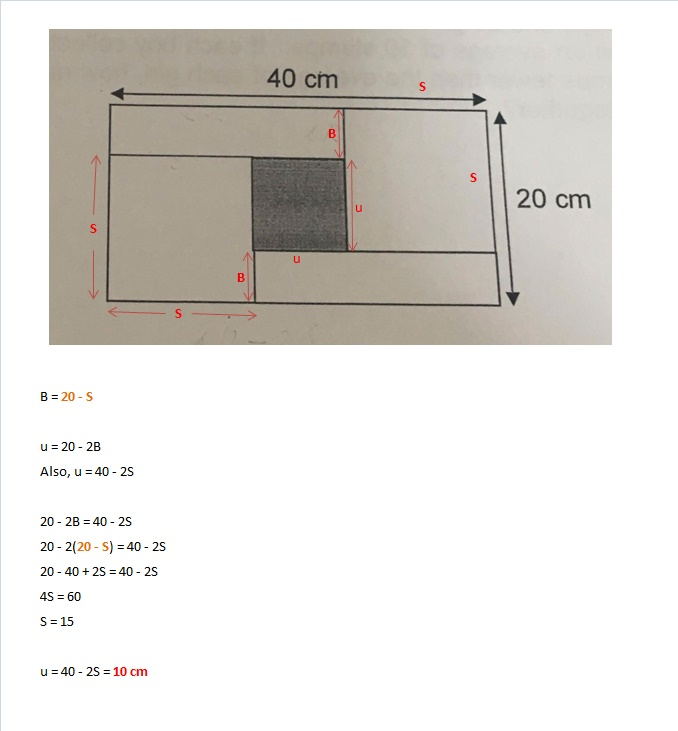# QuestionCut the big rectangle into half in the middle.

Each red stroke is the same unit = 20/4=5cm

length of shaded square=2 red strokes =10cm

0 Replies 2 Likes

length of identical rectangle + side of unshaded square ——- 40
length of identical rectangle – breadth of identical rectangle = 40 – 20 = 20

2 x (side of unshaded square – breadth of identical rectangle) ——- 40 – 20 = 20
side of unshaded square – breadth of rectangle ——- 20/2 = 10

length of identical rectangle – side of unshaded square ——- 20 – 10 = 10
10 + side of unshaded square + side of unshaded square ——- 40
side of unshaded square ——- (40  – 10)/2  = 15
side of shaded square ——- 40 – 15 – 15 = 10
or
breadth of identical rectangle ——- 20 – 15 or 25 – 20 = 5
20 – 5 – 5 =10

Ans : 10 cm.

0 Replies 1 Like0 Replies 1 Like